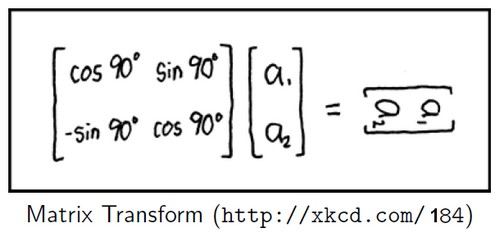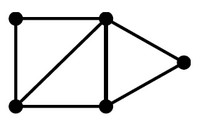# Linear Algebra - Matrix

The Traditional notion of a matrix is:

• a two-dimensional array
• a rectangular table

of known or unknown numbers

One simple role for a matrix: packing together a bunch of columns or rows

## Example

Matrix $A_(i,j)$ of $A[i,j]$

$j=@$ $j=\#$ $j=\%$
$i=a$ 1 2 3
$i=b$ 4 5 6

where:

Index Type In Matrix A
i rows 2 rows [1,2,3] and [4,5,6]
j columns 3 columns: [1,4], [2,5] and [3,6]

Rows and columns are vectors:

• Row ’a’ is the vector [1,2,3]
• Column '%' is the vector [3,6]

## Order

The size, or order, of a matrix is given by identifying the number of rows and columns.

The order of matrix A is 2×3

## Function

Matrix as a function: An R x C Matrix over the field F is a function from R x C to F where:

• R is finite set of unique Row labels: {a, b}
• C is finite set of unique Column labels: {@, #, %}

As it's a function it can be interpreted as an R x C-vector:

From matrix to function: $f (x) = M * x$ where M is a matrix

If M is an R x C matrix over $\mathbb{F}$ then

• domain of f is $\mathbb{F}^C$
• co-domain of f is $\mathbb{F}^R$

Example of function:

• Rotation
• Translation

### Example

#### Example 1

Let M be the matrix $\begin{array}{r|rrr} & \# & @ & ? \\ \hline a & 1 & 2 & 3 \\ b & 10 & 20 & 30 \end{array}$ and define $f ({\bf x}) = M * {\bf x}$ then:

• domain of f is $\mathbb{F}^{\#,@,?}$
• co-domain of f is $\mathbb{F}^{a,b}$

f maps $\begin{array}{rrr} \# & @ & ? \\ \hline 2 & 2 & -2 \\ \end{array}$ to $\begin{array}{rr} a & b \\ \hline 0 & 0 \\ \end{array}$

#### Example 2

Define $f({\bf x}) = \begin{bmatrix} 1 & 2 & 3 10 & 20 & 30 \end{bmatrix} * {\bf x}$ :

• domain of f is $\mathbb{R}^3$
• co-domain of f is $\mathbb{R}^2$

f maps [2, 2,-2] to [0,0]

## Dimension

For the following function

• $f : \mathbb{F}^A \rightarrow \mathbb{F}^B$
• also noted as $f : \mathbb{F}^n \rightarrow \mathbb{F}^m$
• $f ({\bf x}) = M * {\bf x}$

we know:

• Since the domain is $\mathbb{F}^A$ , that the input x is an A-vector.
• Since the co-domain is $\mathbb{F}^B$ , that the output is a B-vector
• then that M must be a B x A matrix

## Operations

### Transpose

Transpose swaps rows and columns. See Dimensional Data Operation - (Pivot|Transpose|Cross-tab|Matrix)

$$\begin{bmatrix} \begin{array}{rrr} 4 & 1 & -3 \\ 2 & 2 & -2 \end{array} \end{bmatrix}^T = \begin{bmatrix} \begin{array}{rr} 4 & 2 \\ 1 & 2 \\ -3 & -2 \end{array} \end{bmatrix}$$

$$\begin{bmatrix} \begin{array}{r} 4 \\ 1 \\ -3 \end{array} \end{bmatrix}^T = \begin{bmatrix} \begin{array}{rrr} 4 & 1 & -3 \end{array} \end{bmatrix}$$

$$\begin{bmatrix} \begin{array}{rr} 4 & 2 \\ 1 & 2 \\ -3 & -2 \end{array} \end{bmatrix}^T = \begin{bmatrix} \begin{array}{rrr} 4 & 1 & -3 \\ 2 & 2 & -2 \\ \end{array} \end{bmatrix}$$

### Inverting

Only square matrices can be inverted, and square matrices are not guaranteed to have an inverse. If the inverse exists, then multiplying a matrix by its inverse will produce the identity matrix.

Theorem: The transpose of an invertible matrix is invertible.

If $A$ has an inverse $A^{-1}$ then $AA^{-1}$ is identity matrix

Converse: If BA is identity matrix then A and B are inverses? Not always true.

Theorem: Suppose A and B are square matrices such that BA is an identity matrix 1. Then A and B are inverses of each other.

Matrices A and B are inverses of each other if and only if both AB and BA are identity matrices.

$$A B = A A^{-1} = I_n$$

A invertible matrix has an inverse.

Corollary

Let A be an R x C matrix. Then A is Linear Algebra - Function (Set) if and only if |R| = |C| and the columns of A are linearly independent.

Proof: Let $\mathbb{F}$ be the field. Define $f : \mathbb{F}^C \mapsto \mathbb{F}^R$ by $f (x) = Ax$ Then A is an invertible matrix if and only if f is an invertible function.

The function f is invertible

• iff dim Ker f = 0 and dim $\mathbb{F}^C$ = dim $\mathbb{F}^R$
• iff nullity A = 0 and |C| = |R|.

nullity A = 0

• iff dim Null A = 0
• iff Null A = {0}
• iff the only vector x such that Ax = 0 is x = 0
• iff the columns of A are linearly independent

Example:

• $\begin{bmatrix}1 & 2 & 3 \\ 4 & 5 & 6\end{bmatrix}$ is not square and so cannot be invertible.
• $\begin{bmatrix}1 & 2 \\ 3 & 4 \end{bmatrix}$ is square and its columns are linearly independent so it is invertible.
• $\begin{bmatrix}\begin{array}{r|r|r}1 & 1 & 2 \\ 2 & 1 & 3 \\ 3 & 1 & 4 \end{array}\end{bmatrix}$ is square but its columns are not linearly independent so it is not invertible.

## Null Space

Null space of a matrix A (Written Null A) is: $$\{u : A * u = 0\}$$

## Nullity

The nullity of matrix A is the dimension of the Null Space written:

• dim Null A

## Type

A vector can be:

### Identity

D x D identity matrix is the matrix $1_D$ such that $1_D [k, k] = 1 \text{ for all } k \in D$ and zero elsewhere.

Often letter I (for “identity”) is used instead of 1

$$\mathbf{I_n} = \begin{bmatrix} 1 & 0 & 0 & \dots & 0 \\\ 0 & 1 & 0 & \dots & 0 \\\ 0 & 0 & 1 & \dots & 0 \\\ \vdots & \vdots & \vdots & \ddots & \vdots \\\ 0 & 0 & 0 & \dots & 1 \end{bmatrix}$$

def identity(D): return Mat((D,D), {(k,k):1 for k in D})


### Diagonal

$\begin{bmatrix} d_1 & & \\ & \ddots & \\ & & d_3 \end{bmatrix}$

Let $d_1, \dots , d_n$ be real numbers. Let $f : \mathbb{R}^n \rightarrow \mathbb{R}^n$ be the function such that $f ([x_1, \dots , x_n]) = [d_1*x_1, \dots , d_n*x_n]$ .

For a domain D, a D x D matrix M is a diagonal matrix if M[r , c] = 0 for every pair $r, c \in D$ such that $r \neq c$ .

A matrix is called a diagonal matrix when the only entries allowed to be nonzero form a diagonal

### Column-orthogonal

If columns of a matrix are orthonormal then it's a column-orthogonal matrix (should be called orthonormal ….)

### Orthogonal

If a matrix is square and column-orthogonal, it's an orthogonal matrix

## Property

### Rank

For a matrix M:

• the row rank of M is the rank of its rows,
• and the column rank of M is the rank of its columns.

Equivalently, the row rank of M is the dimension of Row M, and the column rank of M is the dimension of Col M.

Consider the matrix $$M = \begin{bmatrix} 1 & 0 & 0 \\ 0 & 2 & 0 \\ 2 & 4 & 0 \end{bmatrix}$$ where:

• The rows are the vectors: [1, 0, 0], [0, 2, 0], [2, 4, 0]. The set of these vectors has rank two, so the row rank of M is two.
• The columns of M are [1, 0, 2], [0, 2, 4], and [0, 0, 0]. Since the third vector is the zero vector, it is not needed for spanning the column space. Since each of the first two vectors has a nonzero where the other has a zero, these two are linearly independent, so the column rank is two.

Rank Theorem: For every matrix M, row rank equals column rank.

Lemma: For any matrix A, row rank of A  column rank of A

### Sparse

A sparse matrix has many positions with a value of zero.

Systems designed to efficiently support sparse matrices look a lot like databases: They represent each cell as a record (i,j,value).

The benefit is that you only need one record for every non-zero element of a matrix.

For example, the matrix

 0 2 -1 1 0 0 0 0 -3 0 0 0

can be represented as a table

row # (i) column # (j) value
0 1 2
0 2 -1
1 0 1
2 2 -3

## Operations

### Multiplication

Two matrices may be added or subtracted only if they are of the same order.

Recommended PagesGeometry - Rotation

Rotation is a geometric transformation and can be applied through the following transformation matrix Identity: Rotation matrixGeometry - Transformation Matrix

A geometric transformation can be represented by a matrix. THE advantage of using transformation matrices is that cumulative transformations can be described by simply multiplying the matrices that describe...Graph - Data Structure (Physical Representation)

A graph is represented generally in a physical data structure The graph is composed of two set. a set of vertices (node) Node a b c and set of egde represented for a : directed graph...Linear Algebra

“Linear” “algebra” is the branch of mathematics: concerning vector spaces, often finite or countably infinite dimensional, as well as linear mappings between such spaces. Such an investigation...Linear Algebra - (Matrix|QR) Factorization

matrices can be written as the product of matrices in special form Matrix factorizations are useful mathematically and computationally: Mathematical: They provide insight into the nature of matrices—each...Linear Algebra - Basis of a Vector Space

A basis for vector space V is a linearly independent set of generators for V. Thus a set S of vectors of V is a basis for V if S satisfies two properties: Property B1 (Spanning) Span S = V, and Property...Linear Algebra - Column Vector (One-column matrix)

A vector can be (seen|interpreted) as a one-column matrix. To get a one-row matrix, use . Multiplying a matrix A by a one-column matrix B: By matrix-vector definition of matrix-matrix multiplication,...Linear Algebra - Dimension of a vector space

The dimension of a vector space V is the size of a basis for that vector space written: dim V. rank If U is a subspace of W then D1: (or ) and D2: if then Example: Suppose V = Span...Linear Algebra - Linear Dependency

Vectors v1, . . . , vn are linearly dependent if the zero vector can be written as a nontrivial linear combination of the vectors: In this case, we refer to the linear combination as a linear dependency...Linear Algebra - Linear Function (Weighted sum)

f is a linear function if she is defined by where: M is an R x C matrix and A Linear function can be expressed as a matrix-vector product: If a function can be expressed as a matrix-vector productvector...